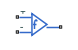# Band-Limited Op-Amp

Model band-limited operational amplifier

• Library:
• Simscape / Electrical / Integrated Circuits

•## Description

The Band-Limited Op-Amp block models a band-limited operational amplifier. If the voltages at the positive and negative ports are Vp and Vm, respectively, the output voltage is:

where:

• A is the gain.

• Rout is the output resistance.

• Iout is the output current.

• s is the Laplace operator.

• f is the 3-dB bandwidth.

The input current is:

`$\frac{{V}_{p}-{V}_{m}}{{R}_{in}}$`

where Rin is the input resistance.

The block does not use the initial condition you specify using the Initial output voltage, V0 parameter if you select the Start simulation from steady state check box in the Simscape™Solver Configuration block.

## Ports

### Conserving

expand all

Electrical conserving port associated with the op-amp non-inverting input

Electrical conserving port associated with the op-amp inverting input

Electrical conserving port associated with the op-amp output voltage

## Parameters

expand all

The open-loop gain of the operational amplifier.

The resistance at the input of the operational amplifier that the block uses to calculate the input current.

The resistance at the output of the operational amplifier that the block uses to calculate the drop in output voltage due to the output current.

The lower limit on the operational amplifier no-load output voltage.

The upper limit on the operational amplifier no-load output voltage.

The maximum positive or negative rate of change of output voltage magnitude.

The open-loop bandwidth, that is, the frequency at which the gain drops by 3 dB compared to the low-frequency gain, A.

The output voltage at the start of the simulation when the output current is zero.

Note

This parameter value does not account for the voltage drop across the output resistor.

## Version History

Introduced in R2008a